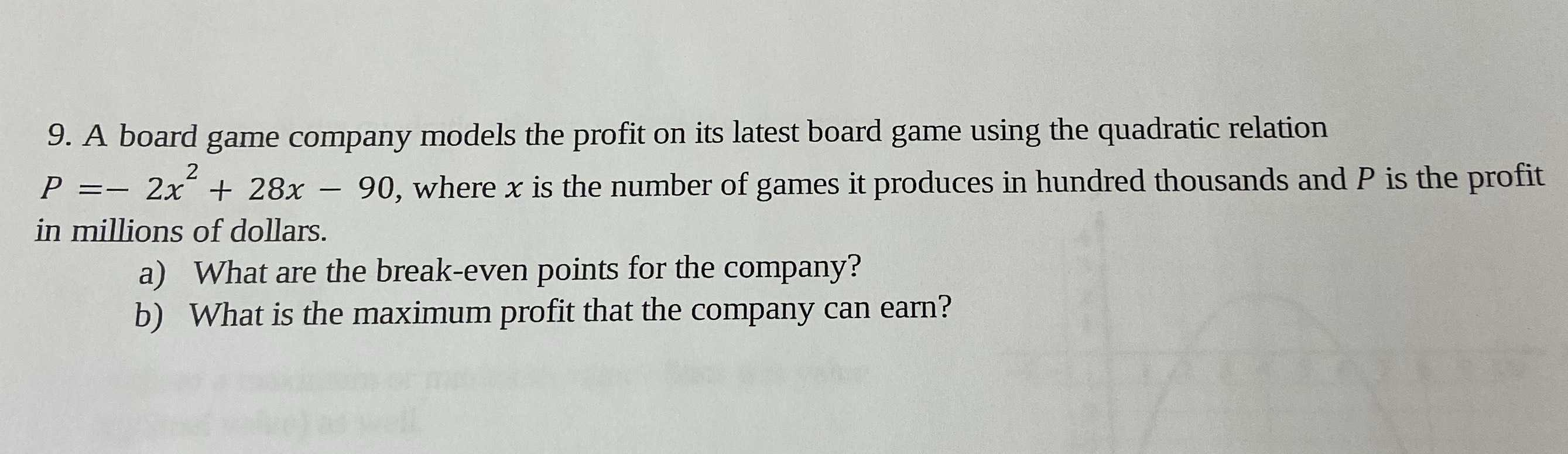### ¿Todavía tienes preguntas de matemáticas?

Pregunte a nuestros tutores expertos
Algebra
Pregunta9. A board game company models the profit on its latest board game using the quadratic relation

$$P = - 2 x ^ { 2 } + 28 x - 90 ,$$ where $$x$$ is the number of games it produces in hundred thousands and $$P$$ is the profit in millions of dollars.

a) What are the break-even points for the company?

b) What is the maximum profit that the company can earn?

(a)$$- 2x^{2} + 28x- 90= 0$$

(x-9)(x-5)=0

x1=9,x2=5

(b)The vertex coordinates of the parabola are$$（- \frac{b}{2a},\frac{4ac- b^{2} }{4a} ）$$

$$- \frac{b}{2a} = 7$$

$$- 2\times 7^{2} + 28\times 7- 90= 8$$

max:8 millions of dollars

Solución
View full explanation on CameraMath App.